• 最小费用最大流问题 解决如下最小费用最大流问题。 查了很多资源发现用MATLAB操作的好用的不多，所以综合了一下很多资源，给出了自己的理解。 基本思路为：把各条弧上单位流量的费用当做距离，用Floyd算法求最...
最小费用最大流问题 解决如下最小费用最大流问题。 以前的资源由于matlab版本问题等已不适用。现在做出修改，适用于matlab2014a以后的版本。 注意，数据格式按代码中的例子的格式，否则需要修改代码。 查了很多资源发现用MATLAB操作的好用的不多，所以综合了一下很多资源，给出了自己的理解。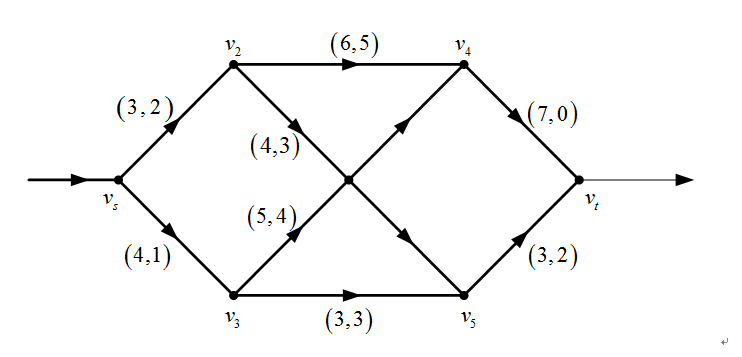基本思路为：把各条弧上单位流量的费用当做距离，用Floyd算法求最短路，确定一条自V1至Vn的最短路；再将这条最短路作为初始路径，用求解最大流问题的方法将其上的流量增至最大可能值；而这条最短路上的流量增加后，其上各条弧的单位流量的费用要重新确定，如此多次迭代，最终得到最小费用最大流。 代码由GreenSim原创（源代码下载积分46），在其基础上加调试。读者根据自己需要设置流量矩阵和费用矩阵。 、代码
展开全文MATLAB 最小费用 最大流 Flod
•  在最大流的有关定义的基础上，若每条有向边除权数c(e)（表示边容量）外还有另外一个权数w(e)（表示单位流所需费用），并且已求得该网络的最大流值为F， 那么最小费用最大流问题，显然可用以下线性规划模型加以描述...

◆ 最小费用最大流

【MCMF问题及数学模型】
在介绍最大流问题时，我们列举了一个最大物资输送流问题。如果这个问题的已知条件还包括每条边运送单位物资的费用，那么怎样运送，才能得到最大运输量，并且输送费用最少？这便是所谓最小费用最大流问题。 　　在最大流的有关定义的基础上，若每条有向边除权数c(e)（表示边容量）外还有另外一个权数w(e)（表示单位流所需费用），并且已求得该网络的最大流值为F， 那么最小费用最大流问题，显然可用以下线性规划模型加以描述：
Min ∑ w(e)f(e) e∈E
满足 0≤f(e)≤c(e)　　，对一切e∈E f+(v)=f-(v)　　　，对一切v∈V 　　 f+(x)=F　　　　　　（最大流约束） 　　　　　　　　 （或f-(y)=F )
【算法思路】
解决最小费用最大流问题，一般有两条途径。一条途径是先用最大流算法算出最大流，然后根据边费用，检查是否有可能在流量平衡的前提下通过调整边流量，使总费用得以减少？只要有这个可能，就进行这样的调整。调整后，得到一个新的最大流。 　  然后，在这个新流的基础上继续检查，调整。这样迭代下去，直至无调整可能，便得到最小费用最大流。这一思路的特点是保持问题的可行性（始终保持最大流），向最优推进。另一条解决途径和前面介绍的最大流算法思路相类似，一般首先给出零流作为初始流。这个流的费用为零，当然是最小费用的。然后寻找一条源点至汇点的增流链，但要求这条增流链必须是所有增流链中费用最小的一条。如果能找出增流链，则在增流链上增流，得出新流。将这个流做为初始流看待，继续寻找增流链增流。这样迭代下去，直至找不出增流链，这时的流即为最小费用最大流。这一算法思路的特点是保持解的最优性（每次得到的新流都是费用最小的流），而逐渐向可行解靠近（直至最大流时才是一个可行解）。 　　由于第二种算法和已介绍的最大流算法接近，且算法中寻找最小费用增流链，可以转化为一个寻求源点至汇点的最短路径问题，所以这里介绍这一算法。在这一算法中，为了寻求最小费用的增流链，对每一当前流，需建立伴随这一网络流的增流网络。例如图 1 网络G 是具有最小 费用的流，边旁参数为c(e) , f(e) , w(e)，而图 2 即为该网络流 的增流网络G′。增流网络的顶点和原网络相同。 按以下原则建 立增流网络的边：若G中边(u，v)流量未饱，即f(u,v) ＜ e(u,v)，则G ' 中建边(u,v)，赋权w ' (u,v)=w(u,v)；若G中边(u, v)已有流量，即f(u,v)〉0，则G′中建边(v,u)，赋权w′(v,u) =-w(u,v)。建立增流网络后，即可在此网络上求源点至汇点的最短路径，以此决定增流路径，然后在原网络上循此路径增流。这里，运用的仍然是最大流算法的增流原理，唯必须选定最小费用的增流链增流。     计算中有一个问题需要解决。这就是增流网络G ′中有负权边，因而不能直接应用标号法来寻找x至y的最短路径，采用其它计算有负权边的网络最短路径的方法来寻找x至y的最短路径，将 大大降低计算效率。为了仍然采用标号法计算最短路径，在每次建立增流网络求得最短路径后,可将网络G的权w(e)做一次修正，使再建的增流网络不会出现负权边，并保证最短路径不至于因此而改变。下面介绍这种修改方法。 当流值为零，第一次建增流网络求最短路径时，因无负权边，当然可以采用标号法进行计算。为了使以后建立增流网络时不出现负权边，采取的办法是将 G中有流边（f(e)＞0）的权w(e)修正为0。为此， 每次在增流网络上求得最短路径后，以下式计算G中新的边权w " (u,v)：
w " (u,v)=L(u)-L(v)+w(u,v) (*)
式中 L(u),L(v) -- 计算G′的x至y最短路径时u和v的标号值。第一次求最短径时如果(u,v)是增流路径上的边， 则据最短 路径算法一定有 L(v)=L(u)+w ' (u,v)=L(u)+w(u,v)， 代入(*)式必有
w″(u,v)=0。
如果(u,v)不是增流路径上的边，则一定有： 　　　 L(v)≤L(u)+w(u,v)， 代入(*)式则有 w(u,v)≥0。
可见第一次修正w(e)后，对任一边，皆有w(e)≥0， 且有流 的边（增流链上的边），一定有w(e)=0。以后每次迭代计算，若 f(u,v)＞0，增流网络需建立(v,u)边，边权数w ' (v,u)=-w(u,v) =0，即不会再出现负权边。     此外，每次迭代计算用(*)式修正一切w(e)， 不难证明对每一条x至y的路径而言，其路径长度都同样增加L(x)-L(y)。因此，x至y的最短路径不会因对w(e)的修正而发生变化。
【计算步骤】
１. 对网络G=[V,E,C,W]，给出流值为零的初始流。 ２. 作伴随这个流的增流网络G′=[V′,E′,W′]。 G′的顶点同G：V′=V。 若G中f(u,v)＜c(u,v)，则G′中建边(u,v)，w(u,v)=w(u,v)。 若G中f(u,v)＞0，则G′中建边(v,u)，w′(v,u)=-w(u,v)。 ３. 若G′不存在x至y的路径，则G的流即为最小费用最大流， 停止计算；否则用标号法找出x至y的最短路径P。 ４. 根据P，在G上增流： 对P的每条边(u,v)，若G存在(u,v)，则(u,v)增流；若G存在(v,u)，则(v,u)减流。增（减）流后，应保证对任一边有c(e)≥ f(e)≥0。 ５. 根据计算最短路径时的各顶点的标号值L(v)，按下式修 改G一切边的权数w(e):
L(u)-L(v)+w(e)→w(e)。
６. 将新流视为初始流，转２。


展开全文网络 算法 c
• 基于matlab2016的最小费用最大流问题求解，内含增广链路函数[path,value] = AugmentingPath(G,s,t)和一个demo函数。 寻找增广链路时，使用了matlab自带的最短路径shortestpath函数，demo中使用了matlab自带的...
• 实验三使用matlab求解最小费用最大流问题 精品文档 精品文档 收集于网络如有侵权请联系管理员删除 收集于网络如有侵权请联系管理员删除 精品文档 收集于网络如有侵权请联系管理员删除 北京联合大学 实验报告 项目...
• 实 验 三 使 用 ma t l a b 求 解 最 小 费 用 最 大 流 算 问题 精品文档 北京联合大学 实验报告 项目...5 月 6 日 收集于网络如有侵权请联系管理员删除 精品文档 实验三使用 matlab 求解最小费用最大流问题 一实
• ## Java实现最小费用最大流问题

万次阅读 多人点赞 2019-07-26 17:48:33
最大流有多组解时，给每条边在附上一个单位费用的量，问在满足最大流时的最小费用是多少？ 2 解决方案 下面代码所使用的测试数据如下图： package com.liuzhen.practice; import java.util.ArrayList; import ...
1 问题描述 在最大流有多组解时，给每条边在附上一个单位费用的量，问在满足最大流时的最小费用是多少？
2 解决方案 下面代码所使用的测试数据如下图：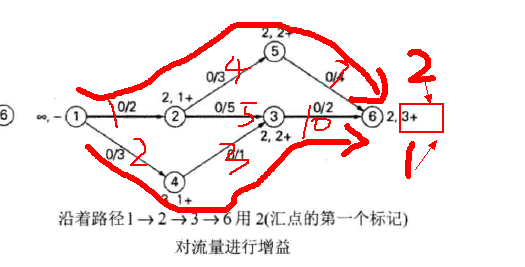package com.liuzhen.practice;

import java.util.ArrayList;
import java.util.Scanner;

public class Main {
public static int MAX = 1000;
public static int n;   //图中顶点数目
public static boolean[] used = new boolean[MAX];   //判断顶点是否在队列中
public static int[] pre = new int[MAX];   //记录最短增广路径中相应节点的前节点
public static int[] distance = new int[MAX];   //记录源点到图中其他所有顶点的最短距离
public static int[] capacity = new int[MAX];  //用于记录遍历图每一次得到增广路径的流量
public static ArrayList<edge>[] map;   //图的邻接表
//表示图中边信息内部类
static class edge {
public int from;   //边的起点
public int to;     //边的终点
public int cap;    //边的容量
public int cost;   //边的费用

public edge(int from, int to, int cap, int cost) {
this.from = from;
this.to = to;
this.cap = cap;
this.cost = cost;
}
}
//输入给定图数据
@SuppressWarnings("unchecked")
public void init() {
Scanner in = new Scanner(System.in);
n = in.nextInt();
int k = in.nextInt();  //给定图的边数目
map = new ArrayList[n];
for(int i = 0;i < n;i++)
map[i] = new ArrayList<edge>();
for(int i = 0;i < k;i++) {
int from = in.nextInt();
int to = in.nextInt();
int cap = in.nextInt();
int cost = in.nextInt();
map[from].add(new edge(from, to, cap, cost));  //正向边
map[to].add(new edge(to, from, 0, -cost));     //反向边
}
}

//寻找顶点start到顶点end的最短路径（PS：即费用最少的一条增广路径）
public boolean spfa(int start, int end) {
int[] count = new int[n];
for(int i = 0;i < n;i++) {
used[i] = false;
pre[i] = -1;
distance[i] = Integer.MAX_VALUE;
capacity[i] = Integer.MAX_VALUE;
}
used[start] = true;
pre[start] = start;
distance[start] = 0;
count[start]++;
ArrayList<Integer> list = new ArrayList<Integer>();
while(!list.isEmpty()) {
int index = list.get(0);
list.remove(0);
used[index] = false;
for(int i = 0;i < map[index].size();i++) {
edge temp = map[index].get(i);
if(temp.cap > 0 && distance[temp.to] > distance[index] + temp.cost) {
//记录顶点start到图中其它顶点之间的最短费用距离
distance[temp.to] = distance[index] + temp.cost;
pre[temp.to] = index;
//记录增广路径能够流通的最大流量
capacity[temp.to] = Math.min(capacity[index], temp.cap);
if(!used[temp.to]) {
used[temp.to] = true;
count[temp.to]++;
if(count[temp.to] > n)   //用于判断图中是否有负环
return false;
}
}
}
}
if(distance[end] != Integer.MAX_VALUE && pre[end] != -1)
return true;
return false;
}

public int getResult() {
init();   //输入给定图数据
int minCost = 0;
int start = 0;   //把源点设置为顶点0
int end = n - 1;  //把汇点设置为顶点n - 1
while(true) {
if(spfa(start, end) == false)
break;
System.out.println("增广路径增量："+capacity[end]+", 费用流："+distance[end]);
minCost += distance[end] * capacity[end];
int last = end;
int begin = end;
System.out.print("汇点出发");
while(begin != start) {
last = begin;
begin = pre[last];
int i = 0, j = 0;
System.out.print("——>"+last);
for(;i < map[begin].size();i++) {
if(map[begin].get(i).to == last)
break;
}
map[begin].get(i).cap -= capacity[end];  //正向边剩余流量减少
for(;j < map[last].size();j++) {
if(map[last].get(j).to == begin)
break;
}
map[last].get(j).cap += capacity[end];  //反向边剩余流量增加
}
System.out.println("——>"+begin);
}
return minCost;
}

public static void main(String[] args) {
Main test = new Main();
int result = test.getResult();
System.out.println(result);
}
}

运行结果：
7
1 2 1
3 3 2
2 5 5
4 3 4
5 2 10
2 1 3
5 4 7
增广路径增量：2, 费用流：12
汇点出发——>5——>4——>1——>0
增广路径增量：1, 费用流：15
汇点出发——>5——>2——>3——>0

展开全文Java 最小费用 最大流
• 博文链接https://blog.csdn.net/weixin_43428682/article/details/94600364。 正确代码链接...这个代码有一点问题，不需要下载。查看我的博客中提供的链接，那个好用。适用于2014a以后的版本
• Bellman-Ford、SPFA、改进的Dijkstra三种算法解决最小费用最大流问题以及相关代码的实现。
摘要
今日，对最小费用最大流问题进行了一个简单的研究，并针对网上的一些已有算法进行了查找和研究。博客和资料很多，但是留下的算法很多运行失败、出错，或者意义不明。这里，个人对其中的Bellman-Ford、SPFA、改进的Dijkstra三种应用于最小费用最大流的算法进行了实现，经过测试，确保其可行性。
网络流
关于网络流，这里引入几个概念：
源点：有n个点，有m条有向边，其中有一个点比较特殊，它只出不进，即入度为0。这样的点我们称为源点，一般用字母S表示。汇点：另一个点也比较特殊，只进不出，即出度为0。这样的点我们称为汇点，一般用字母T表示。容量和流量：每条有向边上有两个量，容量和流量，从i到j的容量表示为c[i,j]表示，流量则用f[i,j]表示。
通常来说，我们可以将这些边具象成道路，流量就是这条道路上的车的容量，容量就是道路可以承受的最大的车流量。 很明显，流量≤容量。 而对于每个不是源点和汇点的节点而言，可以类比为没有存储功能的货物的中转站。所有进入他们的流量等于所有从它出来的流量。
最大流：把源点比作工厂的话，问题就是求工厂最大可以发出多少货物，而不至于超过道路的容量限制，也即，最大流问题。
求解思路： 首先，假如所有边上额流量都不超过容量，那么我们就把这一组流量，或者说，这个流，称为一个可行流。 一个最简单的可行流的例子就是零流，即所有的流量都是0的流。
我们从这个零流开始考虑，假如有这样一条路径，这条路从原点开始一直一段一段的连接，就可以到达汇点。并且，这条路径上的每一段都会满足流量＜容量（注意，不是≤，而是严格＜）那么，我们就一定可以找到这条路径上的每一段的（容量-流量）的值中的最小值delta。我们把这条路上的每一段的流量都加上这个delta，就一定可以保证目前这个流依然是可行流。这样，我们就找到了一个更大的流，它的流量是之前的流量+delta，而这条路径就称为增广路径。我们不断地从起点开始寻找增广路径，每次都对其进行增广，直到源点和汇点不再连通，也就是找不到增广路径为止。当我们找不到增广路径时，当前的流量就是最大流。
补充：
寻找增广路径的时候我们可以简单的从原点开始做BFS，并不断修改这条路上的delta量，直到找到汇点或者找不到增广路径为止。在程序实现的过程中，我们通常只是使用一个c数组来记录容量，而不是记录流量。当流量+delta时，我们可以通过容量-delta来实现，以方便程序编写。
增加反向边的目的： 在做增广路径时可能会阻塞后来的增广路径，换计划说，做增广路径本来是有一个顺序的，只有按照有这一顺序，才能知道最大流。 但是我们在寻找时是任意的，为了修正，我们就每次讲流量加入到了反向弧中从而让后面的流能够进行自我的调整。
例子：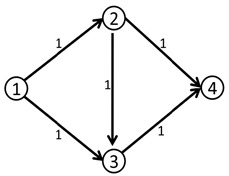我们第一次，可以找到1-2-3-4这条增广路径，这条路径上的delta值显然为1。
此时，我们在修改之后得到了下面这个流。其中，边上的数字代表流量。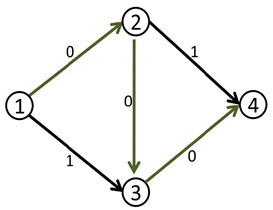此时，边（1,2）和边（3,4）上的边就等于容量了，我们也再也找到不到其他的增广路径，于是，当前的流量是1。
然而，这个答案并不是最大流，因为我们可以同时走1-2-4和1-3-4，这样，可以得到流量为2的最大流。
之所以出现这样的问题，是因为我们在路径寻找的过程中，没有给一个“后悔”的机会，应该有一个不走2-3-4而改走2-4的机制。
而解决这个问题的办法，就是利用一种叫做反向边的概念来解决。 即每条边(i, j)都会有一条反向边(j,i)，反向边也同样有它的容量。
在第一次找到增广路径之后，在把路径上每一段容量减少delta的同时，也把每一段上的反方向的容量增加delta。
c[x,y]-=delta; c[y,x]+=delta;
就上面这个例子，当我们找到1-2-3-4这条增广路径之后，将容量修改如下：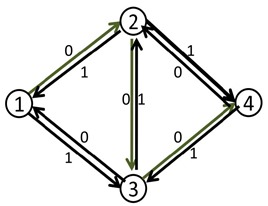此时我们再去寻找增广路径，就可以得到一条：1-3-2-4，将这条路径增广之后，得到最大流为2.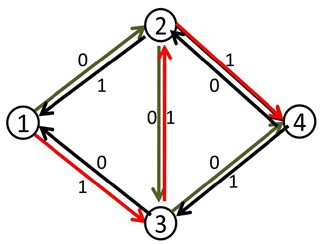这样为何有效？ 实际上，当我们第二次的增广路径走3-2这条反向边时，就相当于把2-3这条正向边已经用的流量给“退”了回去，不走2-3这条路，从而改走从2点出发的其他的路径也即是2-4。
而如果这里没有2-4怎么办？ 这时，假如没有2-4这条道路，那么最终这条增广路径在生成过程中也不会存在，因为最终它根本无法到达汇点。 同时，本来在3-4上的流量则是由1-3-4来“接管”。而最终2-3这条路径正向流量为1，反向流量也为1，等于没有流。
最小费用最大流
对一个费用容量网络，具有相同流量f的可行流中，总费用最小的可行流称为该费用容量网络关于流量f的最小费用流。简称为流量为f的最小费用流。
什么是最小费用最大流问题： 给定网络D=(V,A,C) 每一条弧(vi,vj)上，除了已给容量Cij外，还给了一个单位流量的费用b(vi,vj)>=0. 所谓最小费用最大流问题就是求一个最大流f，使流的总输送费用最小。
对于例子：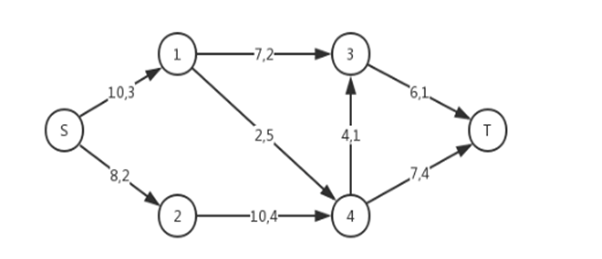从S出发，到达T，正确路径结果，为：
S->1->3->TS->2->4->3->TS->2->4->5->T
最大流为10，最小费用为84。 以下算法在该图中测试，均可得出正确结果。
贝尔曼-福特算法（Bellman-Ford algorithm）
贝尔曼-福特算法（Bellman-Ford algorithm），是求解单元最短路径的一种算法。 它的基本原理是对图进行|V| - 1次松弛操作，得到所有可能的最短路径。 它比Dijkstra算法好的部分在于，在计算最短路径的班的权值可以为负，实现起来比较简单。 缺点则是时间复杂度较高，为O(|V||E|)。不过算法已经有了一些改进方案，比如队列优化的Bellmanford算法（SPFA算法），一定程度上提高了效率。
算法原理： 贝尔曼-福特算法与迪科斯彻算法类似，都以松弛操作为基础，即估计的最短路径值渐渐地被更加准确的值替代，直至得到最优解。 在两个算法中，计算时每个边之间的估计距离值都比真实值大，并且被新找到路径的最小长度替代。 然而，迪科斯彻算法以贪心法选取未被处理的具有最小权值的节点，然后对其的出边进行松弛操作；而贝尔曼-福特算法简单地对所有边进行松弛操作，共**|V| - 1**次。 在重复地计算中，已计算得到正确的距离的边的数量不断增加，直到所有边都计算得到了正确的路径。 因为算法可以使用负权值的边，因此贝尔曼-福特算法比迪科斯彻算法适用于更多种类的输入。
优化选项：
循环的提前跳出 在实际操作中，贝尔曼-福特算法经常会在未达到|V|-1前就给出解，|V|-1就是最大值。因此可以在循环中设置判定，在某次循环不再松弛时，直接退出循环，进行负权环判定。队列优化 队列优化的贝尔曼-福特算法——SPFA算法基本思路与原算法是一样的，不过该算法的提升在于它不会盲目尝试所有的节点，而是维护一个备选节点队列，并且仅有节点被松弛之后才会放入到队列中。
代码实现：
#include "stdafx.h"
#include <iostream>
#include <algorithm>
#include <map>
#include <math.h>
#include <stdio.h>
#include <string.h>
#include <algorithm>
#include <vector>
#include <queue>
#include <stack>

#define MAXN 5050
#define INF 0x3f3f3f3f

using namespace std;

int n, m, s, t;
int u, v, c, w;
int maxFlow, minCost;

struct Edge
{
int from, to, flow, cap, cost;
};

bool vis[MAXN];
int p[MAXN], a[MAXN], d[MAXN];
vector<int> g[MAXN];
vector<Edge> edges;

void init(int n)
{
for (int i = 0; i <= n; i++)
g[i].clear();
edges.clear();
}
void addedge(int from, int to, int cap, int cost)
{
Edge temp1 = { from, to, 0, cap, cost };
Edge temp2 = { to, from, 0, 0, -cost };//允许反向增广
edges.push_back(temp1);
edges.push_back(temp2);
int len = edges.size();
g[from].push_back(len - 2);
g[to].push_back(len - 1);
}

//贝尔曼-福特算法实现
bool bellmanford(int s, int t)
{
for (int i = 0; i < MAXN; i++)
d[i] = INF;
d[s] = 0;
memset(vis, false, sizeof(vis));
memset(p, -1, sizeof(p));
p[s] = -1;
a[s] = INF;
queue<int> que;
que.push(s);
vis[s] = true;
while (!que.empty())
{
int u = que.front();
que.pop();
vis[u] = false;
for (int i = 0; i < g[u].size(); i++)
{
Edge& e = edges[g[u][i]];
if (e.cap > e.flow&&d[e.to] > d[u] + e.cost)//进行松弛，寻找最短路径也就是最小费用
{
d[e.to] = d[u] + e.cost;
p[e.to] = g[u][i];
a[e.to] = min(a[u], e.cap - e.flow);
if (!vis[e.to])
{
que.push(e.to);
vis[e.to] = true;
}
}
}
}
if (d[t] == INF)
return false;
maxFlow += a[t];
minCost += d[t] * a[t];
for (int i = t; i != s; i = edges[p[i]].from)
{
edges[p[i]].flow += a[t];
edges[p[i] ^ 1].flow -= a[t];
}
return true;
}

void MCMF()
{
while (bellmanford(s, t))
continue;
return;
}

int _tmain(int argc, _TCHAR* argv[])
{

cout << "节点数为："; cin >> n;
cout << "边数为："; cin >> m;
cout << "源点编号为："; cin >> s;
cout << "汇点编号为："; cin >> t;

cout << "输入 " << m << " 条边的信息：" << endl;
while (m--)
{
cout << "起点："; cin >> u;
cout << "终点："; cin >> v;
cout << "容量："; cin >> c;
cout << "费用："; cin >> w;
cout << "-----------------" << endl;
}

MCMF();
cout << "最大流为：" << maxFlow << endl;
cout<< "最小费用为"<<minCost << endl;
cout << endl;

system("pause");
return 0;

}

SPFA算法
算法描述：
初始化：distance数组（从源点s到各点的最小费用）全部赋值为inf，用一个队列保存所有待松弛的顶点，初始时将s点放入队列中。队列+松弛操作：每次出队一个顶点u，对其所有的边进行松弛，如果存在某条边u->v松弛成功（dist(v)>dist(u)+w(u,v)），则将v加入队列中（当v不在队列时）；重复以上操作直到队列为空或者发现负权环。 如果网络中存在负权回路，则算法永远都不会结束，陷入死循环。
判断是否存在负权环的方法： 对任何一个顶点，每进入一次队列，意味着需要进行一次松弛，即如果某个顶点进入队列的次数超过V，说明存在负权环。
算法步骤：
建立一个队列，将源点加入队列中，建立一个数组dist记录源点到所有点的最短路径（初始为inf，源点到本身的最短路径是0）。从队列中取出队头元素，刷新其连接的所有点的最短路径；如果刷新成功且被刷新点不在队列中，则把该点加入到队尾。重复执行以上步骤直到队列为空或者队列中存在负权环。
代码实现：
#include "stdafx.h"
#include<cstdio>
#include<cstring>
#include<algorithm>
#include<queue>
#include<iostream>

#define MAXN 5050

using namespace std;

bool vis[MAXN];
int n, m, s, t;
int u, v, c, w;
int cost[MAXN], pre[MAXN], last[MAXN], flow[MAXN];
int maxFlow, minCost;
struct Edge
{
int from, to, flow, cost;
}edge[MAXN];

queue <int> q;

void addedge(int from, int to, int flow, int cost)
{
edge[num_edge].to = to;
edge[num_edge].flow = flow;
edge[num_edge].cost = cost;

edge[num_edge].to = from;
edge[num_edge].flow = 0;
edge[num_edge].cost = -cost;

}

bool SPFA(int s, int t)
{
memset(cost, 0x7f, sizeof(cost));
memset(flow, 0x7f, sizeof(flow));
memset(vis, 0, sizeof(vis));
q.push(s); vis[s] = 1; cost[s] = 0; pre[t] = -1;

while (!q.empty())
{
int now = q.front();
q.pop();
vis[now] = 0;
for (int i = head[now]; i != -1; i = edge[i].from)
{
if (edge[i].flow>0 && cost[edge[i].to]>cost[now] + edge[i].cost)
{
cost[edge[i].to] = cost[now] + edge[i].cost;
pre[edge[i].to] = now;
last[edge[i].to] = i;
flow[edge[i].to] = min(flow[now], edge[i].flow);
if (!vis[edge[i].to])
{
vis[edge[i].to] = 1;
q.push(edge[i].to);
}
}
}
}
return pre[t] != -1;
}

void MCMF()
{
while (SPFA(s, t))
{
int now = t;
maxFlow += flow[t];
minCost += flow[t] * cost[t];
while (now != s)
{
edge[last[now]].flow -= flow[t];
edge[last[now] ^ 1].flow += flow[t];
now = pre[now];
}
}
}

int _tmain(int argc, _TCHAR* argv[])
{

cout << "节点数为："; cin >> n;
cout << "边数为："; cin >> m;
cout << "源点编号为："; cin >> s;
cout << "汇点编号为："; cin >> t;

cout << "输入 " << m << " 条边的信息：" << endl;
while (m--)
{
cout << "起点："; cin >> u;
cout << "终点："; cin >> v;
cout << "容量："; cin >> c;
cout << "费用："; cin >> w;
cout << "-----------------" << endl;
}

MCMF();
cout << "最大流为：" << maxFlow << endl;
cout << "最小费用为：" << minCost << endl;
cout << endl;

system("pause");
return 0;
}

改进的Dijkstra算法
算法描述： 用于求解指定两点间的最短路，或从指定点到其余个点的最短路。是目前求非负权网络最短路问题的最好方法。 基本步骤：
将所有的顶点分成两个集合，P集合和Q集合，初始时P集合只有源点，其他顶点都在Q集合中。每次选择P集合中新加入的顶点u，用该顶点作为中转点更新Q集合中的顶点的最短路（松弛）；选择Q中最短路值最小的顶点加入到集合P中。重复步骤2直到集合Q中没有顶点。
由于最小费用最大流网络中存在负权值，Dijkstra算法不能直接求解最小费用最大流问题，如果最小费用最大流网络中的权值都非负，则可使用Dijkstra算法。引入势函数h(u)为上一次Dijkstra算法的dist(u)（表示从源点到顶点u的最短距离），对每一条边（u,v）,h(v)<=h(u)+w(u,v)成立，则下一次计算中dist(v)=dist(u)+w(u,v)+h(u)-h(v)，所有的dist值必然都大于等于0，则可以继续用Dijkstra算法求解最短路。
代码实现：
#include "stdafx.h"
#include <iostream>
#include <algorithm>
#include <queue>
#include <math.h>
#include <stdio.h>
#include <string.h>
#include <algorithm>
#include <functional>

#define MAXN 5050
#define INF 0x3f3f3f3f
#define P pair<int,int>

using namespace std;

struct edge
{
int to, cap, cost, rev;
};

int n, m, s, t;
int u, v, c, w;
int maxFlow, minCost;

vector<edge> G[MAXN];
int h[MAXN];
int dist[MAXN], prevv[MAXN], preve[MAXN];

void addedge(int from, int to, int cap, int cost)
{
edge temp1 = { to, cap, cost, (int)G[to].size() };
edge temp2 = { from, 0, -cost, (int)G[from].size() - 1 };
G[from].push_back(temp1);
G[to].push_back(temp2);
}

//Dijkstra算法实现
void MCMF(int s, int t, int f)
{
fill(h + 1, h + 1 + n, 0);
while (f > 0)
{
priority_queue<P, vector<P>, greater<P> > D;
memset(dist, INF, sizeof dist);
dist[s] = 0; D.push(P(0, s));
while (!D.empty())
{
P now = D.top(); D.pop();
if (dist[now.second] < now.first) continue;
int v = now.second;
for (int i = 0; i<(int)G[v].size(); ++i)
{
edge &e = G[v][i];
if (e.cap > 0 && dist[e.to] > dist[v] + e.cost + h[v] - h[e.to])
{
dist[e.to] = dist[v] + e.cost + h[v] - h[e.to];
prevv[e.to] = v;
preve[e.to] = i;
D.push(P(dist[e.to], e.to));
}
}
}
if (dist[t] == INF) break;
for (int i = 1; i <= n; ++i) h[i] += dist[i];
int d = f;
for (int v = t; v != s; v = prevv[v])
d = min(d, G[prevv[v]][preve[v]].cap);
f -= d; maxFlow += d;
minCost += d * h[t];
for (int v = t; v != s; v = prevv[v])
{
edge &e = G[prevv[v]][preve[v]];
e.cap -= d;
G[v][e.rev].cap += d;
}
}
}

int _tmain(int argc, _TCHAR* argv[])
{

cout << "节点数为："; cin >> n;
cout << "边数为："; cin >> m;
cout << "源点编号为："; cin >> s;
cout << "汇点编号为："; cin >> t;

cout << "输入 " << m << " 条边的信息：" << endl;
while (m--)
{
cout << "起点："; cin >> u;
cout << "终点："; cin >> v;
cout << "容量："; cin >> c;
cout << "费用："; cin >> w;
cout << "-----------------" << endl;
}

MCMF(s, t, INF);

cout << "最大流为：" << maxFlow << endl;
cout << "最小费用为" << minCost << endl;
cout << endl;

system("pause");
return 0;
}

算法对比
名称特点不足Bellman-Ford可以解决负权边，但不允许有负环每次循环值均对所有元素进行松弛判断，造成许多不必要的操作。SPFA进阶版的BF，使用队列进行优化，每次循环值选择当前节点相邻的若干节点进行松弛。在稀疏图上十分高效。单路增广。SPFA需要维护较为复杂的标号和队列操作，同时为了修正标号，需要不止一次地访问某些节点，速度会比较慢。改进的Dijkstra速度普遍比SPFA要快。无法直接处理负权边图，需要对算法进行改进。
补充
除了上述三种算法之外，还有诸如Dinic、ZKW等算法，不过个人没有研究，这里就不再赘述了。
参考文献
 最小费用最大流(详解+模板)  数据结构与算法分析 - 网络流入门（Network Flow）  最小费用最大流问题  维基百科-最小费用最大流问题 【最小费用最大流】知识点讲解  P3381 【模板】最小费用最大流 题解
展开全文• 下面的最小费用最大流算法采用的是基于Floyd最短路算法的Ford和Fulkerson...最大流问题的方法将其上的流量增至最大可能值而这条最短路上的流量增加后其上各条弧的单位流量的费用要重新确定如此多次迭代最终得到最小费用...
• 在网上翻了很久，终于找到一个大概看得懂的证明了。 https://bartholomewa.wordpress.com/2018/04/25/最小费用最大流详解模板/
• 最小费用最大流 网络流的费用： 在实际应用中，与网络流有关的问题，...最小费用最大流问题 给定网络G，要求G的在最大用流flow（就是这个图的最大流）的情况下，使流的总费用最小。 其思想与求最大流的增广路算
• 最小费用最大流（最大流+最短路） 最大费用最大流（最大流+最长路） 最小费用最大流 为什么说最小费用最大流就是最大流+最短路呢， 可以这样想： 有一条流量为f的增广路，对于其中的每一条边都有一个单位费用cost[i...算法 dijkstra
• 最小费用最大流问题 一、方法 对偶法 始终保持网络中的可行流是最小费用流，然后不断调整，是流量逐步增大，最终成为最小费用的最大流 二、定理 若X是流量为f(X)的最小费用流，u是关于X的所有增广链中费用最小的...
• 有向图的最小费用最大流问题 预备知识：最大流问题，增广路算法。 问题说明：把物品从结点s（称为源点）运送至结点t（称为汇点），每条边上有一个二元组（x，y），x表示边的最大运送能力，y表示运送单位物品的花费。...算法
• 最小费用最大流问题叠加算法 例：求解图1的到流量依次为2、8的最小费用流；并求解其最小费用最大流。弧旁数字为（其中b为单位费用函数，c为容量函数，下同）； 图1 给出一种算法求解最小费用最大流问题。 ...算法
• 无向图的最小费用最大流问题 预备知识：最大流问题，增广路算法，有向图的最小费用最大流问题。 问题说明：把物品从结点s（称为源点）运送至结点t（称为汇点），连接结点u和结点v的无向边上有两个二元组（x，y）和...算法...